﻿ Shifting Graphs Up/Down/Left/Right

# SHIFTING GRAPHS UP/DOWN/LEFT/RIGHT

by Dr. Carol JVF Burns (website creator)
Follow along with the highlighted text while you listen!
Note:
$\,f(x)+2\,$ is read aloud as: ‘$\,f\,$ of $\,x\,$ (slight pause) plus $\,2\,$’
$\,f(x+2)\,$ is read aloud as: ‘$\,f\,$ of (slight pause) $\,x\,$ plus $\,2\,$’
In this section, I over-emphasize the pauses, to help you hear the difference.
• PRACTICE (online exercises and printable worksheets)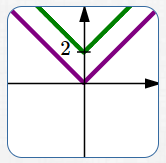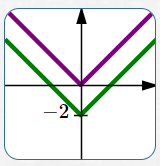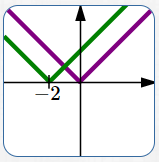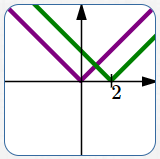$y = f(x)$ $y = f(x) + 2\,$ up $2$ $y = f(x)$ $y = f(x) - 2\,$ down $2$ $y = f(x)$ $y = f(x+2)\,$ left $2$ $y = f(x)$ $y = f(x-2)\,$ right $2$ movements up and down change the $y$-values of points; transformations that affect the $y$-values are intuitive (e.g., to move up $\,2\,$, you add $\,2\,$ to the previous $\,y\,$-value) movements left and right change the $x$-values of points; transformations that affect the $x$-values are counter-intuitive (e.g., to move left $\,2\,$, you replace every $\,x\,$ by $\,x+2\,$, NOT $\,x-2\,$) Shifting up/down/left/right does NOT change the shape of a graph.

The lesson Graphing Tools: Vertical and Horizontal Translations in the Algebra II curriculum
gives a thorough discussion of shifting graphs up/down/left/right.

The key concepts are repeated here.

The exercises in this lesson duplicate those in Graphing Tools: Vertical and Horizontal Translations.

IDEAS REGARDING VERTICAL TRANSLATIONS (MOVING UP/DOWN)
• Points on the graph of $\,y=f(x)\,$ are of the form $\,\bigl(x,f(x)\bigr)\,$.
Points on the graph of $\,y=f(x)+3\,$ are of the form $\,\bigl(x,f(x)+3\bigr)\,$.
Thus, the graph of $\,y=f(x)+3\,$ is the same as the graph of $\,y=f(x)\,$, shifted UP three units.
• Transformations involving $\,y\,$ work the way you would expect them to work—they are intuitive.
• Here is the thought process you should use when you are given the graph of $\,y=f(x)\,$
and asked about the graph of $\,y=f(x)+3\,$:
\begin{align} \text{original equation:} &\quad y=f(x)\cr\cr \text{new equation:} &\quad y=f(x) + 3 \end{align}
$$\begin{gather} \text{interpretation of new equation:}\cr\cr \overset{\text{the new y-values}}{\overbrace{ \strut\ \ y\ \ }} \overset{\text{are}}{\overbrace{ \strut\ \ =\ \ }} \overset{\quad\text{the previous y-values}\quad}{\overbrace{ \strut f(x)}} \overset{\qquad\text{with 3 added to them}\quad}{\overbrace{ \strut\ \ + 3\ \ }} \end{gather}$$
• Summary of vertical translations:
Let $\,p\,$ be a positive number.

 Start with the equation $\,\color{purple}{y=f(x)}\,$. Adding $\,\color{green}{p}\,$ to the previous $\,\color{green}{y}\,$-values gives the new equation $\,\color{green}{y=f(x)+p}\,$. This shifts the graph UP $\,\color{green}{p}\,$ units. A point $\,\color{purple}{(a,b)}\,$ on the graph of $\,\color{purple}{y=f(x)}\,$ moves to a point $\,\color{green}{(a,b+p)}\,$ on the graph of $\,\color{green}{y=f(x)+p}\,$ .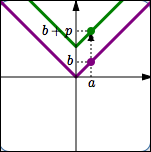Additionally: Start with the equation $\,\color{purple}{y=f(x)}\,$. Subtracting $\,\color{green}{p}\,$ from the previous $\,\color{green}{y}\,$-values gives the new equation $\,\color{green}{y=f(x)-p}\,$. This shifts the graph DOWN $\,\color{green}{p}\,$ units. A point $\,\color{purple}{(a,b)}\,$ on the graph of $\,\color{purple}{y=f(x)}\,$ moves to a point $\,\color{green}{(a,b-p)}\,$ on the graph of $\,\color{green}{y=f(x)-p}\,$.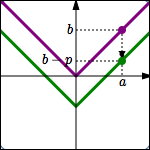This transformation type (shifting up and down) is formally called vertical translation.
IDEAS REGARDING HORIZONTAL TRANSLATIONS (MOVING LEFT/RIGHT)
• Points on the graph of $\,y=f(x)\,$ are of the form $\,\bigl(x,f(x)\bigr)\,$.
Points on the graph of $\,y=f(x+3)\,$ are of the form $\,\bigl(x,f(x+3)\bigr)\,$.
• How can we locate these desired points $\,\bigl(x,f(x+3)\bigr)\,$?

First, go to the point $\,\color{red}{P\bigl(x+3\,,\,f(x+3)\bigr)}\,$ on the graph of $\,\color{red}{y=f(x)}\,$.
This point has the $\,y$-value that we want, but it has the wrong $\,x$-value.

Move this point $\,\color{purple}{3}\,$ units to the left.
Thus, the $\,y$-value stays the same, but the $\,x$-value is decreased by $\,3\,$.
This gives the desired point $\,\color{green}{\bigl(x,f(x+3)\bigr)}\,$.

Thus, the graph of $\,y=f(x+3)\,$ is the same as the graph of $\,y=f(x)\,$, shifted LEFT three units.

Thus, replacing $\,x\,$ by $\,x+3\,$ moved the graph LEFT (not right, as might have been expected!)
• Transformations involving $\,x\,$ do NOT work the way you would expect them to work!
They are counter-intuitive—they are against your intuition.
• Here is the thought process you should use when you are given the graph of $\,y=f(x)\,$
and asked about the graph of $\,y=f(x+3)\,$:
\begin{align} \text{original equation:} &\quad y=f(x)\cr\cr \text{new equation:} &\quad y=f(x+3) \end{align}
$$\begin{gather} \text{interpretation of new equation:}\cr\cr y = f( \overset{\text{replace x by x+3}}{\overbrace{ x+3}} ) \end{gather}$$
Replacing every $\,x\,$ by $\,x+3\,$ in an equation moves the graph $\,3\,$ units TO THE LEFT.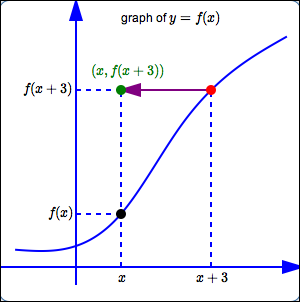• Summary of horizontal translations:
Let $\,p\,$ be a positive number.

 Start with the equation $\,\color{purple}{y=f(x)}\,$. Replace every $\,\color{green}{x}\,$ by $\,\color{green}{x+p}\,$ to give the new equation $\,\color{green}{y=f(x+p)}\,$. This shifts the graph LEFT $\,\color{green}{p}\,$ units. A point $\,\color{purple}{(a,b)}\,$ on the graph of $\,\color{purple}{y=f(x)}\,$ moves to a point $\,\color{green}{(a-p,b)}\,$ on the graph of $\,\color{green}{y=f(x+p)}\,$.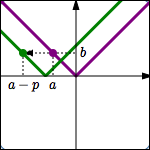Additionally: Start with the equation $\,\color{purple}{y=f(x)}\,$. Replace every $\,\color{green}{x}\,$ by $\,\color{green}{x-p}\,$ to give the new equation $\,\color{green}{y=f(x-p)}\,$. This shifts the graph RIGHT $\,\color{green}{p}\,$ units. A point $\,\color{purple}{(a,b)}\,$ on the graph of $\,\color{purple}{y=f(x)}\,$ moves to a point $\,\color{green}{(a+p,b)}\,$ on the graph of $\,\color{green}{y=f(x-p)}\,$.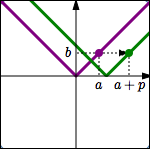This transformation type (shifting left and right) is formally called horizontal translation.
DIFFERENT WORDS USED TO TALK ABOUT TRANSFORMATIONS INVOLVING $\,y\,$ and $\,x\,$

Notice that different words are used when talking about transformations involving $\,y\,$, and transformations involving $\,x\,$.

For transformations involving $\,y\,$
(that is, transformations that change the $\,y$-values of the points), we say:

DO THIS to the previous $\,y$-value.

For transformations involving $\,x\,$
(that is, transformations that change the $\,x$-values of the points), we say:

REPLACE the previous $\,x$-values by $\ldots$

MAKE SURE YOU SEE THE DIFFERENCE!

vertical translations:
going from $\,y=f(x)\,$ to $\,y = f(x) \pm c\,$

horizontal translations:
going from $\,y = f(x)\,$ to $\,y = f(x\pm c)\,$

Make sure you see the difference between (say) $\,y = f(x) + 3\,$ and $\,y = f(x+3)\,$!

In the case of $\,y = f(x) + 3\,$, the $\,3\,$ is ‘on the outside’;
we're dropping $\,x\,$ in the $\,f\,$ box, getting the corresponding output, and then adding $\,3\,$ to it.
This is a vertical translation.

In the case of $\,y = f(x + 3)\,$, the $\,3\,$ is ‘on the inside’;
we're adding $\,3\,$ to $\,x\,$ before dropping it into the $\,f\,$ box.
This is a horizontal translation.

EXAMPLES:
Question:
Start with $\,y = f(x)\,$.
Move the graph TO THE RIGHT $\,2\,$.
What is the new equation?
Solution:
This is a transformation involving $\,x\,$; it is counter-intuitive.
You must replace every $\,x\,$ by $\,x-2\,$.
The new equation is:
$\,y = f(x-2)\,$
Question:
Start with $\,y = x^2\,$.
Move the graph DOWN $\,3\,$.
What is the new equation?
Solution:
This is a transformation involving $\,y\,$; it is intuitive.
You must subtract $\,3\,$ from the previous $\,y\,$-value.
The new equation is:
$\,y = x^2 - 3\,$
Question:
Let $\,(a,b)\,$ be a point on the graph of $\,y = f(x)\,$.
Then, what point is on the graph of $\,y = f(x+5)\,$?
Solution:
This is a transformation involving $\,x\,$; it is counter-intuitive.
Replacing every $\,x\,$ by $\,x+5\,$ in an equation causes the graph to shift $\,5\,$ units to the LEFT.
Thus, the new point is $\,(a-5,b)\,$.
Master the ideas from this section

When you're done practicing, move on to:
horizontal and vertical stretching/shrinking

“Start with $\,y = f(x)\,$.   Move UP $\,2\,$.   What is the new equation?”
“Start with the graph of $\,y = f(x)\,$.   Move this graph UP $\,2\,$.